# Convert Octal to Binary

Problem Statement: Given an Octal Number, convert it into Binary Number.

Examples:

```Example 1:
Input: 345
Output: 011100101
Explanation: Binary equivalent of given Octal expressionis 011100101

Example 2:
Input: 170
Output: 001111000
Explanation: Binary equivalent of given Octal expression is 001111000
```

### Solution

Disclaimer: Don’t jump directly to the solution, try it out yourself first.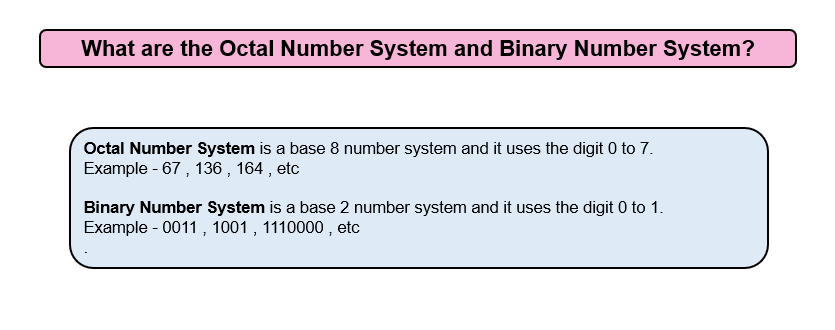Intuition: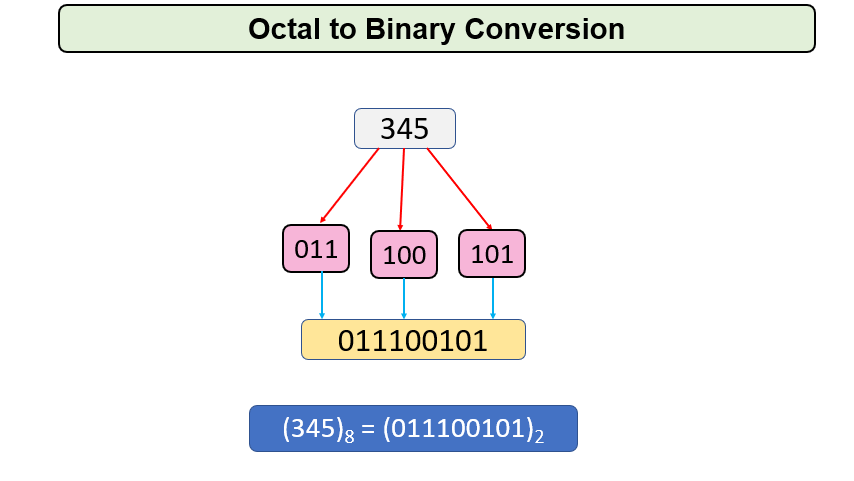Approach

For Octal to Binary Conversion, we will first convert Octal Number System to Decimal Number System and then convert Decimal Number System to Binary Number System.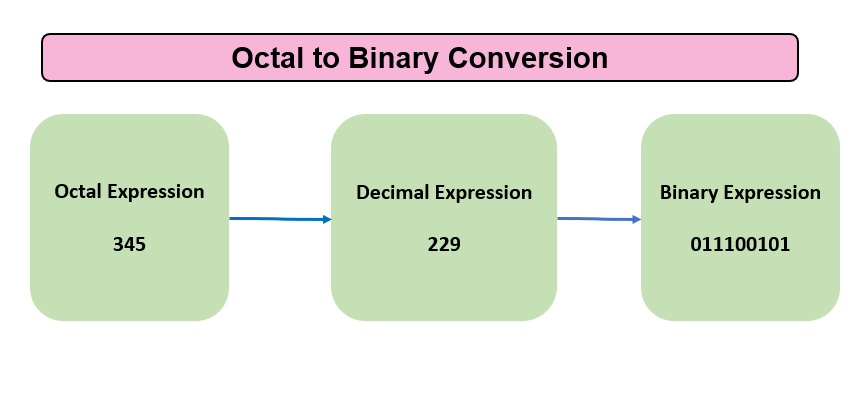For Octal to Decimal Conversion

We will take every digit of the number and multiply it with 8 raised to power i which will increase by 1 when we move to the next digit and then add it to sum. This task is repeated until n becomes 0.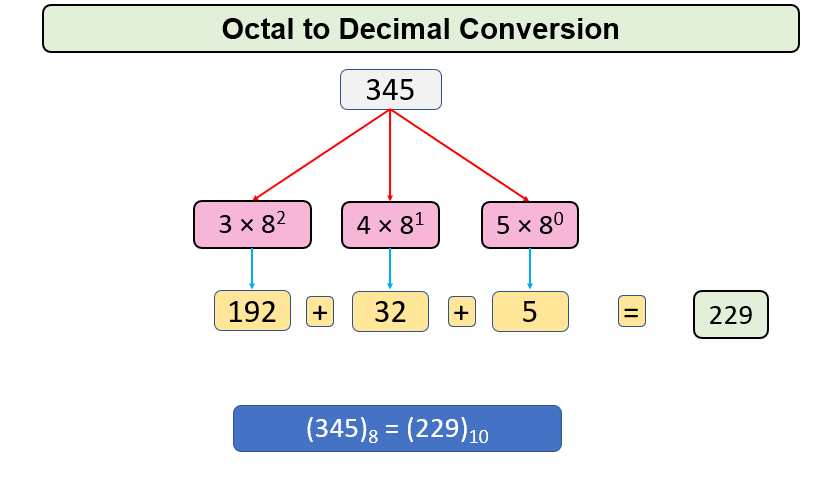For Decimal to Binary Conversion

For Decimal to Binary Conversion, we will divide the given number by 2 ( Since the Binary Numbers System has 2 digits in use ) repeatedly, and its remains will be stored till the number becomes zero.

Let us continue with 229.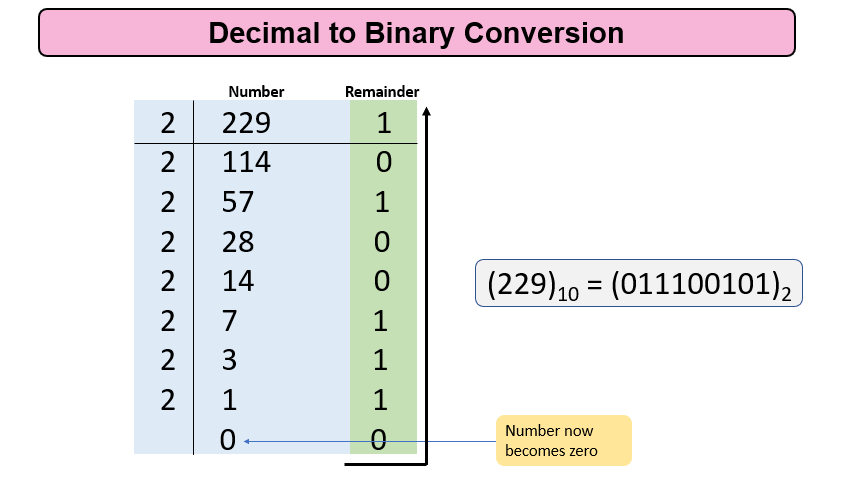Code:

## C++ Code

``````#include <iostream>
#include <math.h>
using namespace std;
int OctaltoDecimal(int Octal)
{
int Decimal = 0;
int i = 0;
while (Octal != 0)
{
int rem = Octal % 10;
Decimal += rem * pow(8, i);
i++;
Octal /= 10;
}
return Decimal;
}
int DecimaltoBinary(int decimal)
{
int Binary = 0;
int i = 0;
while (decimal != 0)
{
int rem = decimal % 2;
Binary += (rem * pow(10, i));
i++;
decimal = decimal / 2;
}
return Binary;
}
int main()
{
int Octal = 345;
int Decimal = OctaltoDecimal(Octal);
cout << "The binary conversion of the given octal number is "<<DecimaltoBinary(Decimal) << endl;
return 0;
}
``````

Output:

The binary conversion of the given octal number is 11100101

Time Complexity: O(log n) where n is the number

Space Complexity: O(1)

## Java Code

``````import java.util.*;
public class Main {
public static int DecimaltoBinary(int decimal) {
int Binary = 0;
int i = 0;
while (decimal != 0) {
int rem = decimal % 2;
Binary += (rem * Math.pow(10, i));
i++;
decimal = decimal / 2;
}
return Binary;
}
public static int OctaltoDecimal(int Octal) {
int Decimal = 0;
int i = 0;
while (Octal != 0) {
int rem = Octal % 10;
Decimal += rem * Math.pow(8, i);
i++;
Octal /= 10;
}
return Decimal;
}
public static void main(String[] args) {
int Octal = 345;
int Decimal = OctaltoDecimal(Octal);
System.out.println("The binary conversion of the given octal number is
"+DecimaltoBinary(Decimal));
}

}

``````

Output:

The binary conversion of the given octal number is 11100101

Time Complexity: O(log n) where n is the number

Space Complexity: O(1)

Special thanks to Gurmeet Singh for contributing to this article on takeUforward. If you also wish to share your knowledge with the takeUforward fam, please check out this article